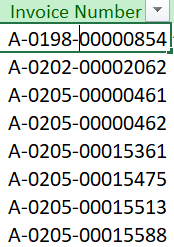Hi All,

i need to add hyper links on each row, img for exampleHow to achieve this.

2. For each row in datatable
3. Assign row(RequiredColumn) = String.Format(“<a href=‘"Url’”>{0}", System.Web.HttpUtility.HtmlEncode(row(RquiredColumn).ToString()))
4. Pass the Url variable name to Url in the above code.
5. If row(RequiredColumn) value is passing in hyperlink it is passing in the ‘{0}’ in the above code.

If this works, Please mention as Solution

Thanks

Hi @darshan.bp ,

can you pls check below one

i have assign cell_counter variable to 1 and incrementiing it.

but check the write cell (=Hyperlink(A2,A2) this one i feel something wrong, how to write so that it automatically increment all the row.

Hi All,
do we have a VBA macro for hyperlinks?

@RAKESH_KUMAR_Tiwari

Try like this

2. Use the following expression in assign `dt.Columns("HL").Expression = "'=HYPERLINK('""[ColumnNameContainingValue]""','""[ColumnNameContainingValue]""')'"`